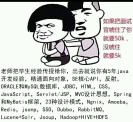# 热榜！万字长文第二篇：深入讲解python中一大数据结构之字典（叮叮当~小葵花课堂开课啦！）

「本文已参与好文召集令活动，点击查看：后端、大前端双赛道投稿，2万元奖池等你挑战！``````在Python程序设计中，字典是一种比较特别的数据类型。它以大括号“{}”包围，并且以“键：值”对的方式# 第一小节课 之 认识字典：

## （1）如何创建并访问字典

### ①创建字典：

``````dict = {'线代': "99", "数据分析": "99", "概率论": "98"}

``````dict1 = {'线代': "99"}
dict2 = {"数据分析": "99"}
dict3 = {"概率论": "98"}

``````知识补给站：

a = {}#### 两个拓展操作——教你如何快速创建一个字典！

``````dict语法：
class dict(**kwarg)
class dict(mapping, **kwarg)
class dict(iterable, **kwarg)

# dict函数作用：通过其他映射（比如其他字典）或者（键，值）对的序列建立字典。

``````dict1 = dict(a='a', b='b', t='t')     # 传入关键字
print(dict1)

dict2 = dict(zip(['one', 'two', 'three'], [1, 2, 3]))   # 映射函数方式来构造字典
print(dict2)

dict3 = dict([('one', 1), ('two', 2), ('three', 3)])    # 可迭代对象方式来构造字典
print(dict3)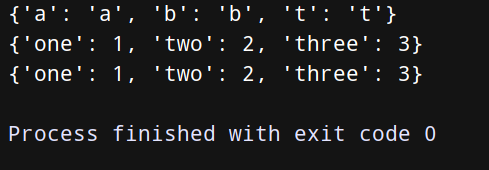``````重点： 使用dict()函数，dict值必须一一对应。
使用dict()函数，必须每个元组里的成比例。才能将元组转成 key:value

``````语法：
dict.fromkeys(seq[, value])

seq -- 字典键值列表。
value -- 可选参数, 设置键序列（seq）的值。

fromkeys() 函数用于创建一个新字典，以序列 seq 中元素做字典的键，value 为字典所有键对应的初始值。

``````dict3 = dict.fromkeys(['name','age'])
print(dict3)

dict4 = dict.fromkeys(['name','age'],10)
print(dict4)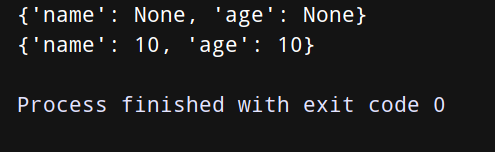需要注意的是：
fromkeys 方法只用来创建新字典，不负责保存。当通过一个字典来调用 fromkeys 方法时，如果需要后续使用一定记得给他复制给其他的变量。

### ②访问字典：

#### 第一阶段：基操勿6！

``````dict = {'线代': "99", "数据分析": "99", "概率论": "98"}      # 创建字典

print('小红同学的线代成绩是：',dict['线代'])                  # 输出线代成绩
print('小红同学的线代成绩是：',dict['数据分析'])               # 输出数据分析成绩
print('小红同学的线代成绩是：',dict['概率论'])                # 输出概率论成绩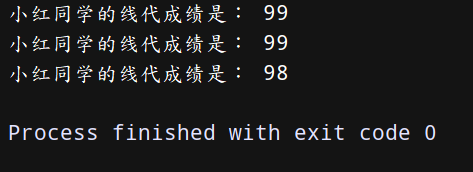#### 第二阶段：项目常用！

（1） get 获取指定key 对应的value，如果key不存在返回none——语法：字典名.get(key)

``````a = {'干干': 11, 'age': 11}
print(a.get('age'))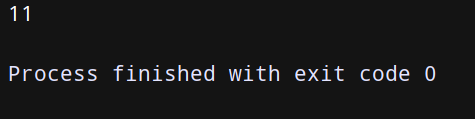（2）keys 取出所有的key——用法：字典名.keys()

``````a = {'干干': 11, 'age': 11}
print(a.keys())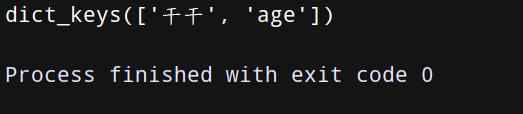（3）values 取出所有的值——用法：字典名.values()

``````a = {'干干': 11, 'age': 11}
print(a.values())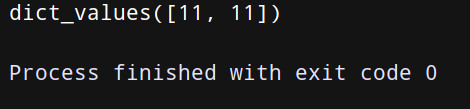（4） items 取出所有的键值对——用法：字典名.items()

``````a = {'干干': 11, 'age': 11}
print(a.items())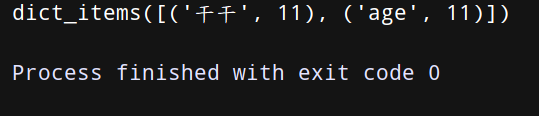（5）setdefault(key) 有此键则查，无则增！

``````di2 = {'name':'干干','age':18,'sex':'男'}
print(di2.setdefault('name'))
di2.setdefault('name2')
print(di2)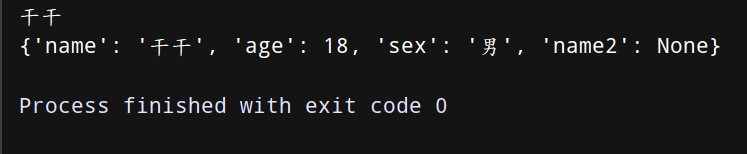### 拓展：如果我们要查某一个键对应的值，而这个键值对在字典中不存在会怎么样呢！

``````dict = {'线代': "99", "数据分析": "99", "概率论": "98"}      # 创建字典

print("dict['语文成绩']:",dict['语文成绩'])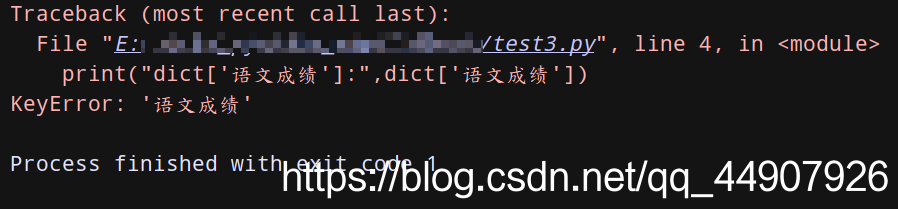## （2）如何添加，修改，删除字典中的元素

``````知识补给站：

### ①字典中添加数据：

#### （1）基操勿6！

``````dict = {'线代': "99", "数据分析": "99", "概率论": "98"}      # 创建字典

dict['数学'] = 100        # 添加字典1
dict['英语'] = 99         # 添加字典2

print(dict)               # 输出字典dict中的值
print('小红同学的数学成绩是：',dict['数学'])     # 显示数学成绩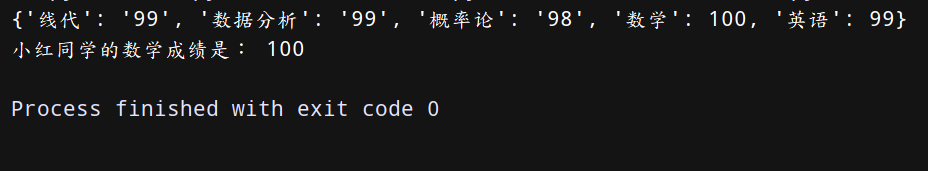``````注意注意注意：
“键值”对的排列顺序与添加顺序不同。因为python不关心键值对的添加顺序，而只关心键和值之间的关联关系！！！

### ②修改字典：

#### （1）基操勿6！

**首先指定字典名，然后使用中括号把要修改的键和新值对应起来。**眼睛亮的同学是不是发现这和字典中添加数据好像一样呢！其实可以很肯定的告诉大家，确实是完全一模一样的，所以可以总结出一句顺口溜：有这个键则修改，无则添加！

``````dict = {'线代': "99", "数据分析": "99", "概率论": "98"}      # 创建字典

dict['线代'] = 100

print('小红同学真正的线代成绩是：',dict['线代'])
print(dict)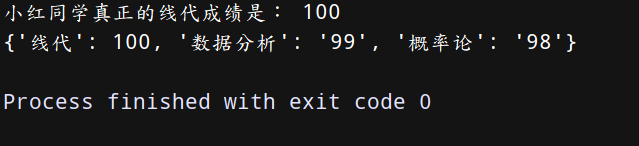#### （2）项目常用！

** update 更新字典、将原字典和新字典整合，key重复的话则新的覆盖老的！**

``````dict = {'name':'干干','age':18,'sex':'男'}
dict.update({'height': 195, 'age': 20})
print(dict)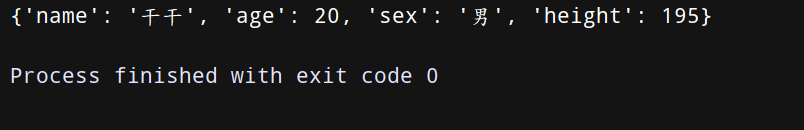### ③删除字典中的数据：

#### （1）基操勿6！

``````dict = {'线代': "99", "数据分析": "99", "概率论": "98"}      # 创建字典

del dict['线代']      # 删除键 '线代'

print(dict)           # 显示字典dict中的元素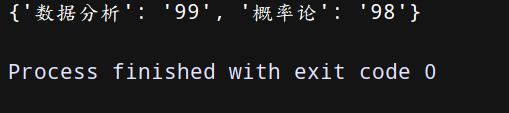#### （2）项目常用！

（1）clear 清空字典——用法：字典名.clear()

``````dict = {'name':'干干','age':18,'sex':'男'}
dict.clear()
print(dict)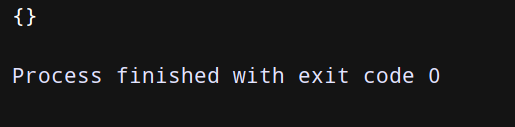（2） pop 弹出指定key的键值对——用法：字典名.pop(key)

``````dict = {'name':'干干','age':18,'sex':'男'}
a = dict.pop('name')
print('弹出的键对应的值为：', a)
print(dict)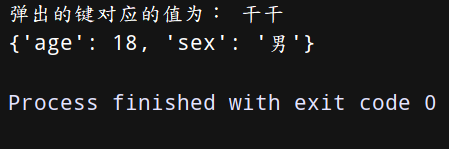（3） popitem 返回并删除字典中的最后一对键和值——用法：字典名.popitem()

``````dict = {'name':'干干','age':18,'sex':'男'}
a = dict.popitem()		# #相当于栈出，不过每次出的是一个键值对

print('删除字典中最后一对键值对：',a)
print(dict)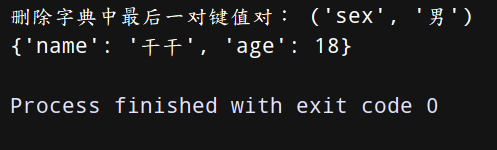# 第二小节课 之 字典的骚操作（项目常用！）：

### ①一键多值字典

``````比如如下字典d和e就是两种典型的一键多值字典，那么，如何使用defaultdict()函数来实现呢？
d = {
'a': [1, 2, 3],
}

e = {
'a': {1, 2, 3},
}

``````from collections import defaultdict
d = defaultdict(list)
d['a'].append(1)
d['a'].append(2)
d['a'].append(3)
print(d)
print(d['a'])		# 字典中的查操作依旧同我们第一小节课讲的那样。

d = defaultdict(set)
print(d)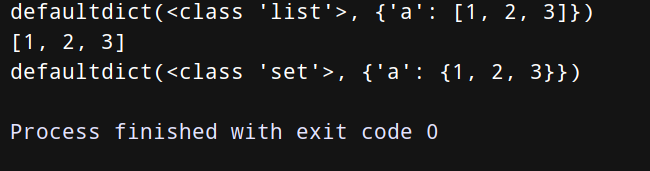但是，我们使用函数defaultdict()会自动创建字典表项以待后面使用。如果不想要这个功能的话，老师现在再教你们一种新的方法：

``````d = {}
d.setdefault('a', []).append(1)
d.setdefault('a', []).append(2)
d.setdefault('b', []).append(3)
print(d)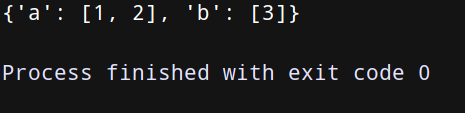``````dict =defaultdict( factory_function)		# defaultdict接受一个工厂函数作为参数

from collections import defaultdict

dict1 = defaultdict(int)
dict2 = defaultdict(set)
dict3 = defaultdict(str)
dict4 = defaultdict(list)
dict1 = 'nice'		#无则增！

# 这样会正常显示dict1字典里key为2对应的value。
print(dict1)

# 我们访问的是通过defaultdict()函数创建的四个字典的key为1对应的值，
# 但是这个key在这四个字典中都并不存在哦，所以返回相应的默认值！
print(dict1)
print(dict2)
print(dict3)
print(dict4)

# 输出：
nice
0
set()

[]

``````知识补给站升级：

from collections import defaultdict
def zero():
return 0
dict = defaultdict(zero)
print(dict)

print(dict['first'])

print(dict)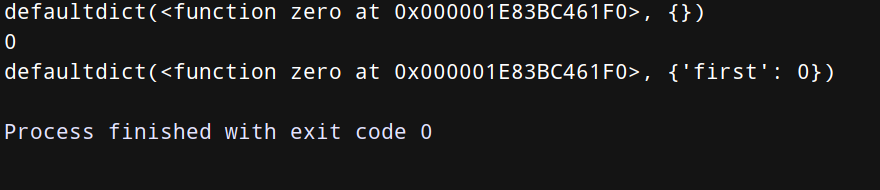### ②获取字典中的最大值和最小值

``````知识补给站：

``````函数zip()语法格式：

zip([iterable,...]

iterable表示一个或多个迭代器。

``````price = {
'小米': 899,
'华为': 1999,
'三星': 3999,
'谷歌': 4999,
'酷派': 599,
'iPhone': 5000,
}

min_price = min(zip(price.values(), price.keys()))		# 获取字典中手机价格最小的手机
print(min_price)

max_price = max(zip(price.values(), price.keys()))		# 获取字典中手机价格最大的手机
print(max_price)

price_sorted = sorted(zip(price.values(), price.keys()))	# 将字典中手机按价格从低到高排序
print(price_sorted)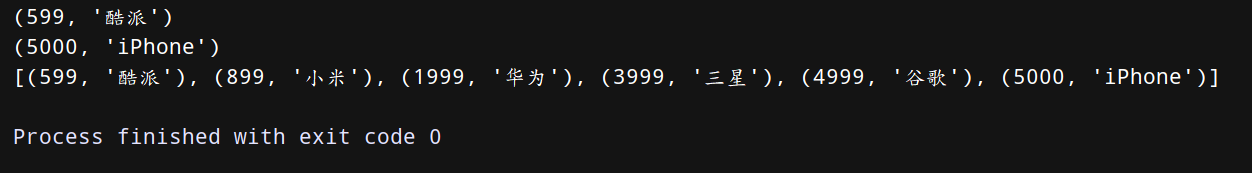``````需要注意的是：

price = {
'小米': 899,
'华为': 1999,
'三星': 3999,
'谷歌': 4999,
'酷派': 599,
'iPhone': 5000,
}

price_and_names = zip(price.values(), price.keys())
print((min(price_and_names)))

print (max(price_and_names))  # 报错error  zip()创建了迭代器，内容只能被消费一次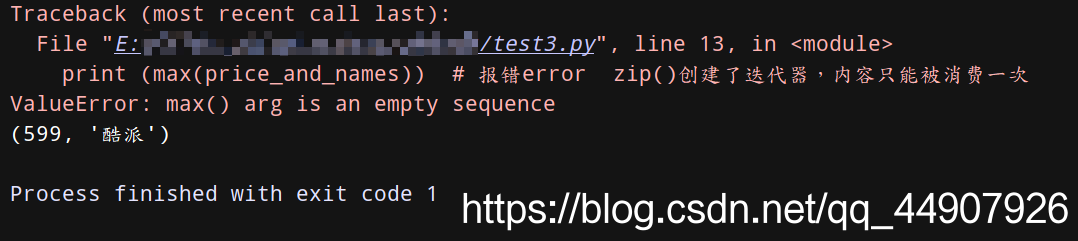``````price = {
'小米': 899,
'华为': 1999,
'三星': 3999,
'谷歌': 4999,
'酷派': 599,
'iPhone': 5000,
}

# 这种直接使用min()和max()函数明显不对哦！这就是按key排序了！
print(min(price))
print(max(price))

print(min(price.values()))
print(max(price.values()))

print(min(price, key=lambda k: price[k]))
print(max(price, key=lambda k: price[k]))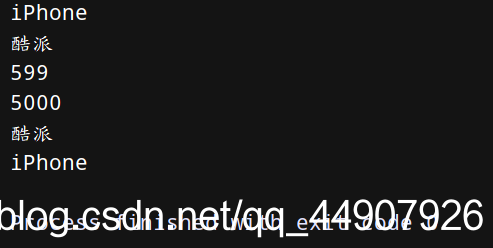#### ③使用字典推导式

``````mcase = {'a': 10, 'b': 34, 'A': 7, 'Z': 3}
mcase_frequency = {
k.lower(): mcase.get(k.lower(), 0) + mcase.get(k.upper(), 0)
for k in mcase.keys()
if k.lower() in ['a','b']
}
print (mcase_frequency)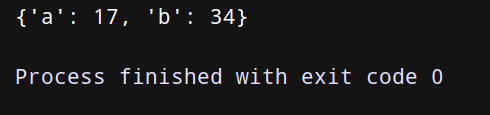``````dict = {'a': 10, 'b': 34}
dict_end = {v: k for k, v in dict.items()}
print(dict_end)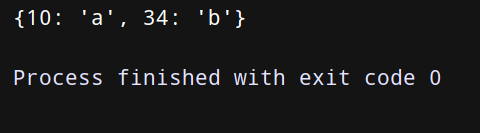``````prices = {'ASP.NET': 49.9, 'Python': 69.9, 'Java': 59.9, 'C语言': 45.9, 'PHP': 79.9}
p1 = {key: value for key, value in prices.items() if value > 50}			# 提取字典prices的子集（value大于50）
print(p1)

tech_names = {'Python', 'Java', 'C语言'}

p2 = {key: value for key, value in prices.items() if key in tech_names}	# 提取字典prices的子集（key存在在集合tech_names里的）
print(p2)

``````prices = {'ASP.NET': 49.9, 'Python': 69.9, 'Java': 59.9, 'C语言': 45.9, 'PHP': 79.9}

tech_names = {'Python', 'Java', 'C语言'}

p3 = dict((key, value) for key, value in prices.items() if value > 50)  # 慢
print(p3)

p4 = {key: prices[key] for key, value in prices.items() if value > 50}  # 慢
print(p4)

### ④获取两个字典中相同的键值对

``````知识补给站：

在python字典中，函数keys()返回keys-view对象，其中显示所有的键。字典中的键可以支持常见的集合操作，

在Python字典中，函数items()返回由键值对组成的items-view对象。这个对象支持类似的集合操作，可以用于

``````a = {
'x': 1,
'y': 2,
'z': 3
}

b = {
'x': 11,
'y': 2,
'w': 10
}
print(a.keys() & b.keys())
print(a.keys() - b.keys())
print(a.items() & b.items())

c = {key: a[key] for key in a.keys() - {'z', 'w'}}	# 使用字典推导式实现，能够修改或过滤掉字典中的内容。
print(c)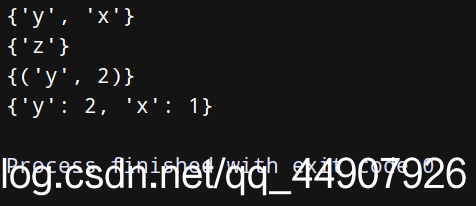### ⑤使用函数itemgetter()对字典进行排序

``````from operator import itemgetter
a = [1,2,3]
b=itemgetter(1)      # 获取对象的第1个域的值
print(b(a))

b=itemgetter(1,0)    # 获取对象的第1个域和第0个的值
print(b(a))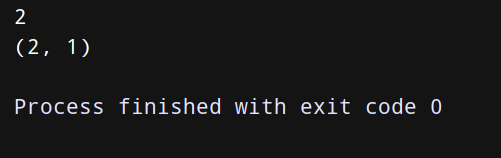``````from operator import itemgetter
rows = [
{'fname': 'AAA', 'lname': 'ZHANG', 'uid': 1001},
{'fname': 'BBB', 'lname': 'ZHOU', 'uid': 1002},
{'fname': 'CCC', 'lname': 'WU', 'uid': 1004},
{'fname': 'DDD', 'lname': 'LI', 'uid': 1003}
]

#分别根据所有字典共有的字段fname和uid进行排序
rows_by_fname = sorted(rows, key=itemgetter('fname'))
rows_by_uid = sorted(rows, key=itemgetter('uid'))
print(rows_by_fname)
print(rows_by_uid)

# itemgetter()函数接收多个键！
rows_by_lfname = sorted(rows, key=itemgetter('lname', 'fname'))
print(rows_by_lfname)

# 使用lambda表达式代替itemgetter()函数的功能！
# 注意：少用lambda表达式，使用itemgetter()函数会运行更快！考虑程序性能问题的话要用itemgetter()函数！
rows_by_fname = sorted(rows, key=lambda r: r['fname'])
rows_by_lfname = sorted(rows, key=lambda r: (r['fname'], r['lname']))
print(rows_by_fname)
print(rows_by_lfname)

# 拓展：itemgetter()函数同样可以用于操作min()和max()函数哦！
print(min(rows, key=itemgetter('uid')))
print(max(rows, key=itemgetter('uid')))

``````输出为：
[{'fname': 'AAA', 'lname': 'ZHANG', 'uid': 1001}, {'fname': 'BBB', 'lname': 'ZHOU', 'uid': 1002}, {'fname': 'CCC', 'lname': 'WU', 'uid': 1004}, {'fname': 'DDD', 'lname': 'LI', 'uid': 1003}]
[{'fname': 'AAA', 'lname': 'ZHANG', 'uid': 1001}, {'fname': 'BBB', 'lname': 'ZHOU', 'uid': 1002}, {'fname': 'DDD', 'lname': 'LI', 'uid': 1003}, {'fname': 'CCC', 'lname': 'WU', 'uid': 1004}]
[{'fname': 'DDD', 'lname': 'LI', 'uid': 1003}, {'fname': 'CCC', 'lname': 'WU', 'uid': 1004}, {'fname': 'AAA', 'lname': 'ZHANG', 'uid': 1001}, {'fname': 'BBB', 'lname': 'ZHOU', 'uid': 1002}]
[{'fname': 'AAA', 'lname': 'ZHANG', 'uid': 1001}, {'fname': 'BBB', 'lname': 'ZHOU', 'uid': 1002}, {'fname': 'CCC', 'lname': 'WU', 'uid': 1004}, {'fname': 'DDD', 'lname': 'LI', 'uid': 1003}]
[{'fname': 'AAA', 'lname': 'ZHANG', 'uid': 1001}, {'fname': 'BBB', 'lname': 'ZHOU', 'uid': 1002}, {'fname': 'CCC', 'lname': 'WU', 'uid': 1004}, {'fname': 'DDD', 'lname': 'LI', 'uid': 1003}]
{'fname': 'AAA', 'lname': 'ZHANG', 'uid': 1001}
{'fname': 'CCC', 'lname': 'WU', 'uid': 1004}

# 那些想要写出我们期末考试中关于字典的选做题的学生好好听了哦——接下来教同学们一些在项目中常用的字典小操作！

## （1）第一个骚操作 之 在字典中同时转换并换算数据

``````知识补给站：

sum(iterable,[,start])

iterable:可迭代对象，如列表。
start:指定相加的参数，如果没有设置默认为0.

>>>sum([0,1,2])
3
>>>sum([0,1,2,3,4],2)		# 列表计算合后再加2
12

``````nums = [1,2,3,4,5]
s = sum(x*x for x in nums)
print(s)
import os
files = os.listdir('.idea')
if any (name.endswith('.py') for name in files):
print('这是一个Python文件!')
s = ('RMB',50,128.88)
print(','.join(str(x) for x in s))

portfolio = [
{'name': 'AAA', 'shares': 50},
{'name': 'BBB', 'shares': 65},
{'name': 'CCC', 'shares': 40},
{'name': 'DDD', 'shares': 35}
]

min_shares = min(s['shares'] for s in portfolio)
print(min_shares)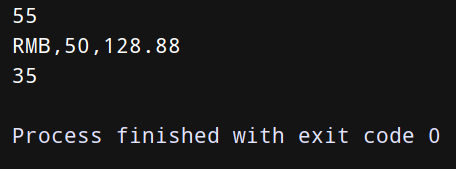## （2）第二个骚操作 之 将多个映射合并为单个映射

``````a = {'x': 1, 'z': 3 }
b = {'y': 2, 'z': 4 }

from collections import ChainMap
c = ChainMap(a,b)

print(c['x']) # Outputs 1 (from a)
print(c['y']) # Outputs 2 (from b)
print(c['z']) # Outputs 3 (from a)

print(len(c))
print(list(c.keys()))
print(list(c.values()))

c['z'] = 10
c['w'] = 40
del c['x']
print(a)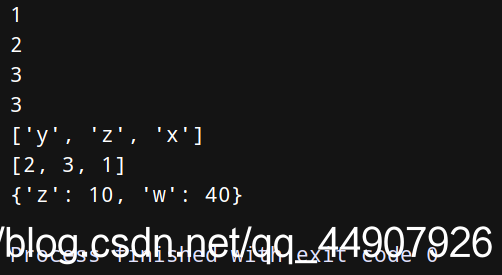## （3）第三个操作 之 创建有序字典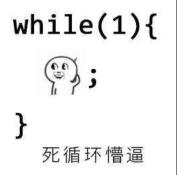``````import collections

dic = collections.OrderedDict()
dic['k1'] = 'v1'
dic['k2'] = 'v2'
dic['k3'] = 'v3'
print(dic)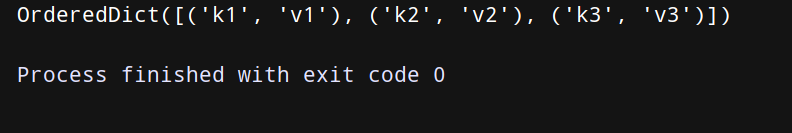``````import collections

dic = collections.OrderedDict()
dic['k1'] = 'v1'
dic['k2'] = 'v2'
dic['k3'] = 'v3'
print(dic.popitem(),dic)
print(dic.popitem(),dic)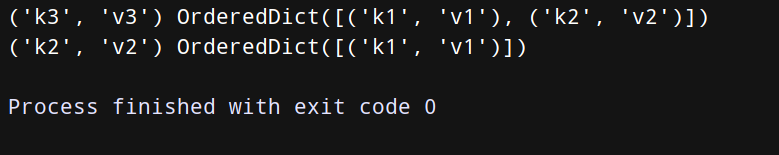``````知识补给站：

10000行内容到OrderedDict列表中），那么需要认真对应用做需求分析，看看因为使用OrderedDict所能带来的好处是否能大过因额

# 结束语——老师历经九九八十一难求取此篇真经，同学们只要潜心研究此经，字典这一知识点必手到擒来！